# An Archaeological Problem

As a first example, consider an MCMC analysis of the model and data described in Naylor and Smith (1988). This concerns inference about five dates forming boundaries between differing, distinct, and abutting periods of pottery production. The data consist of radiocarbon date determinations associated with pottery fragments identified as belonging to one of four periods of pottery production.

Their analysis obtains a posterior density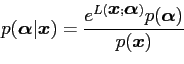(10)

where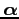is a vector of dates before present2 (BP)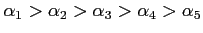, having prior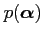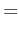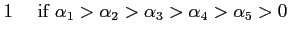(11)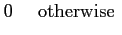(12)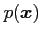is the normalizing constant.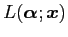is the Log-Likelihood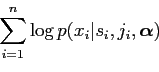(13)

where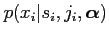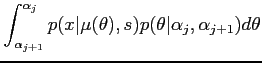(14)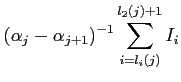(15)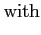(16)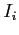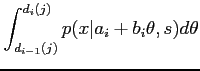(17)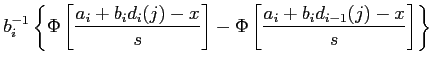(18)being the standard Normal distribution function and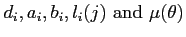are values expressing a piecewise linear radiocarbon calibration curve (Clark, 1979).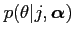(19)(20)

where here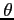is the actual date of manufacture.

The analysis presented here, using MCMC, incorporates into the likelihood a calibration curve, defined by Clark (1979) in piecewise linear segments, relating radiocarbon date to calendar date. This curve includes inversion (i.e. it is not monotonically increasing), which renders its use difficult. Figure 1 shows univariate posterior densities estimated using BKDE for four of the five boundary dates.

Taking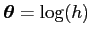(ensuring that the estimate of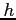at any point is positive) to have prior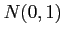, represents a loosely held belief (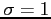) that the density is smooth (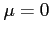), corresponding to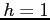. That isin the range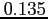to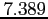being considered probable a priori.

These densities are seen to be considerably less smooth than the corresponding results of Naylor and Smith (1988) who used an older, but smoother, calibration curve. There is some evidence that the likelihood is not smooth and other authors have found that there are considerable problems for maximum likelihood analyses (Clark, 1979; Cunliffe, 1984).

The analysis returns as many points as required. In this example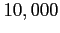points were generated and then reduced to 500 by extracting every 20th result 3. Taking, a uni-dimensional likelihood is constructed with one previous sample.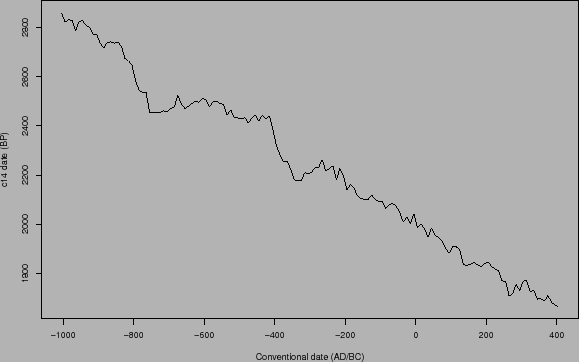In Figure 2 the effect of changing the prior is observable:

1. Priorloosely held belief in a smooth density, (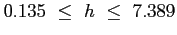).
2. Prior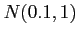loosely held belief in a slightly smoother density (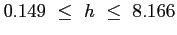).
3. Prior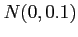stronger belief in a smooth density (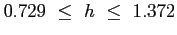).
4. Prior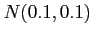stronger belief in a slightly smoother density (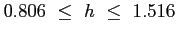).

On initially examining Figure 2, it is apparent that 1 and 2 show the same curve, the weak prior is overridden by the data. In 3 and 4 the curves are similar and smooth, the information in the data being insufficient to override the strong prior.

Having a degree of belief in the confidence placed in an expert opinion'' is central to a subjective Bayesian approach to a problem. Priors arising from either previous knowledge of a process or from some reliable analysis of a situation may be assumed to have some degree of validity. This is expressed here in 3 and 4 by the adoption of a small value for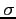leading to the retention of the smooth curve in the density estimate. The larger prior value forin 1 and 2 expresses the opposite, i.e. an assumption that there is no prior belief in a smooth curve and that is reflected in the density estimation obtained. Equally a strong belief in a jagged curve could be imposed, which would seem to be more appropriate here. In all cases, more data reduces the influence of the prior.

Finally, note that the jagged curve in 1 and 2 might be taken to indicate that the curve is under smoothed, however, the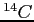calibration curve used here is in nowise linear as can be seen in Figure 3, and this might be reflected in the posterior.

danny 2009-07-23